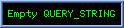Hyperbolic Gaussian Wave Packets

Define Ghypk,la,l, º ò0¥ dcsinh(c))kcosh(c))l (-lca)
For a=2 this is known as a Gaussian Integration.
Note that for large c, ( sinh(c))kcosh(c))l (-lca) » ((k+lc -lca) » (-lca) for a>1.
For small c, ( sinh(c))kcosh(c))l (-lca) » ck(-lca) is guaranteed zero for a<0 but cusps for k<0 , a>0 .
Ghyp0,0,2,l = ½p½l .
Ghyp1,0,2,l = ½ ll-1) òl ½l  dxi (-xi2)
[ Proof :   ò0¥ dc(±c - lc2)   =   (¼l-1) ò0¥ dc(-lc2 ±c - ¼l-1)   =   (¼l-1) ò-/+ ½l¥ dc (-(l½c -/+ ½l)2)
=   (¼l-1) l ò-/+ ½l ¥ dxi (-xi2)     via xi = l½c -/+ ½l with dxi = l½dc  .]

Ghyp1,0,1,l = ½((1-l)-1 - (1+l)-1)  provided l > 1.
[ Proof :   ò0¥ dc(±c - lc)   =   ò0¥ dc((±1 - l)c)   =   -(±1 - l)-1     if l>1 .  .]

For a>1 the (-lca) ensures convergence as t®¥ irrespective of k,l, but k<0 introduces a ck cusp as c®0. For a=1 we require k+l<1.

For a>0 , (-lc-a)  » 1 for large c and tends to 0 as c®0. Ghypk,l,-a,l accordingly diverges as t®¥ unless k+l < 0, providing damping factor (c(k+l)) . k<0 aquires a ck cusp factor at c=0 but this is swamped by the closeness of (-lca) to 0 for small c.

Recall the e4-specific hyperbolic spinor 1-vector representation with time t = x4 º x4x º e4¿x ;     rapidity c º cx,e4 º  cosh-1(x4|x|-1) ;   and 2-blade     x º xx,e4 º xÙe4 = XÙe4 =Xe4     so that for nonnull x
x   =   |x|(-cx~) e4 for nonnull x and x4|X| = |x|2  cosh(csinh(c) = |x|2 ½ sinh(2c) .
Ñxc   =   |x|-2(x4X~ + |X|e4)     timelike and normal to x, with
(Ñxc)2 = Ñx2c = |x|-2     ; x(Ñxc) = x~     ;   X(Ñxc) = |x|-2|X|(x4 + x) .
We have used e4 to denote the timelike direction but the following holds for ÂN-1,1 for any N³2.

To construct e4-specific 1-fields satisfying Ñx¿jx = 0 we observe that Ñx(|X|-m|x|-nx)   =   |X|-m|x|-n(N-n-m - m|X|-2x4XÙe4) so
Ñx¿(|X|-m|x|-nx)   =   0     provided n+m=N .    This motivates the e4-dependant definition
Qm,x º QN,m,x,e4 º |X|-m|x|m-N       for x¹0 with
ÑxQm,x   =   Qm,x(-m|X|-2X + (m-N)|x|-2x) .
ÑXQm,x   =   Qm,x(-m|X|-2 + (m-N)|x|-2)X
Ñx(Qm,xx)   =   -m|X|-m-3|x|m-N x4x~   =   -m|X|-3Qm,x x4x~   =   -m|X|-2Qm,x x4x     which is 0 for m=0 and has zero scalar part for any m.
[ Proof : -m|X|-m-2X|x|-nx + |X|-m(N-n)|x|-n   =   |X|-m|x|-n(-m|X|-2Xx + N-n)  .]
Ñx(Qm,xX)   =   ½Qm,x( N-m-2 + (m-N)(-2cx~) )     , in particular (ÑxQN-2,xX)   =   -QN-2,x(-2cx~)
[ Proof : (ÑxQm,x)X + Qm,x(ÑxX)   =   Qm,x(    -m|X|-2X2 + (m-N)|x|-2xX + (N-1))   =   Qm,x(    N-m-1 + (m-N)|x|-2(|X|2 + x4e4ÙX) )
=   Qm,x(    N-m-1 + (m-N)( sinh(c)2 -  cosh(csinh(c)X~Ùe4 )   =   Qm,x(    N-m-1 + ½(m-N)( cosh(2c)-1  -  sinh(2c)x~ )
=   Qm,x(    ½(N-m) -1 + ½(m-N)( cosh(2c) -  sinh(2c)x~ )   =   ½Qm,x( N-m - 2 + (m-N)(-2cx~) )  .]

Define jx º r|X|-m|x|m-N(-lca) x   =   rQm,x(-lca) x     for scalar l>0 and scaling constant r>0 chosen to normalise the integration of either j4 =  cosh(c)rx = rx4|X|-m|x|m-N(-lca) or rx º |jx| = r|X|-m|x|1+m-N(-lca) according to taste, over a given subspace of interest.

Ñxjx   =   (laca-1-m|X|-2 coth(c) ) rQm,x(-lca) x~   =   (-alca-1 + m|X|-2 coth(c) ) (Ñxc)jx   =   (-alca-1 + m|X|-2 coth(c) ) (Ñxc)Ùjx     has zero scalar part since Ñxc is orthogonal to x and so to jx.
[ Proof : Ñxjx   =   - rlaca-1(-lca)(Ñc)Qm,xx + r( ÑxQm,xx)(-lca)   =   - rQm,xlaca-1(-lca)(e4X)~ - r m|X|-3Qm,xx4(XÙe4)~ (-lca)
=   - rQm,x(-lca) (laca-1 - m|X|-3x4 ) (e4X)~ .     (e4X)~ = (Ñxc)x .  .]

Hence |Ñxjx| = rQm,x (-lca) |laca-1 - m|X|-3x4|
4p|X|2 rx = 4p |X|2-m|x|1+m-N(-lca)
4p|X|2 j4 = 4p t|X|2-m|x|m-N (-lca)

Choosing The Normalisation Constant
Holding |x|=R constant, ò ON-1+-R,0 dN-1x rx   =   roN-1 Rm-N GhypN-1-m,0,a,l independant of R for m=N ,
[ Proof : r ò R¥ dt  oN-1|X|N-2 |X|-m|x|m-N (-lca) oN-1r ò R¥ dt |X|N-2-m|x|m-N (-lca)   =   oN-1r Sm-N ò0¥ dc |X|N-1-m (-lca) since
c/t   =   ((tR-1)2-1)R-1 = (R-2(t2-R2))R-1 = (t2-R2) = |X|-1     Þ dt = |X|dc  .]

Holding t constant, ò We4,te4 dN-1x j4   =   roN-1 GhypN-1-m,0,a,l     independant of t as expected.
[ Proof : c/R = ((tR-1)2-1)(-tR-2) = -t|X|-1R-1     Þ dR = -t-1|X|R dc    ;     ccosh-1(tR-1)    ;     R-1 =  coshct-1
oN-1r t ò 0t dR |X|N-2-m|x|m-N (-lca)   =   oN-1r ò¥0 dc |X|N-1-mR1+m-N (-lca)   =   oN-1r ò0¥ dcsinh(c))N-1-m (-lca)
Within We4,te4, R=|x| varies from maximum t at te4 down to 0 as we cross L+0 and then rises to ¥ outside L+0. Restricting integration to within the forward nullcone corresponds to intergrating R from t down to 0.  .]

Holding t constant, ò We4,te4 dN-1x rx     =   roN-1 GhypN-1-m,-1,a,l     independant of t.
[ Proof : oN-1r ò 0t dR |X|N-2-m|x|1+m-N(-lca)   =   oN-1r t-1 ò0¥ dc |X|N-1-mR2+m-N (-lca)
=   oN-1r t-1 ò0¥ dc  sinh(c)N-1-mR-1 (-lca)   =   oN-1r ò0¥ dc  sinh(c)N-1-m cosh(c)-1 (-lca)  .]

Holding t constant, ò We4,te4 dN-1x rx  tanh(c)   =   roN-1 GhypN-m,-2,a,l     gives the expected e4-speed.
jx = (oN-1 Ghyp0,0,a,l)-1 |X|1-N |x|2 (-lca) x~     for m=N-1 corresponds to an ambiguous  "slow" particle; and
jx = (oN-1 Ghyp-1,0,-a,l)-1 |X|-N |x| (-lc-a) x~     for m=N corresponds to a "near light" particle having near-maximal e4-speed.
In both cases, Ñx¿jx=0 and jx and Ñxjx vanish over L+0 other than at 0 so defining jx=0 " x : x2³0 gives jx nonzero only inside the future nullcone L+0 .

The expected (average) four-velocity is e4 but with a random boost of magnitude propOrtionate to (-lca) where positive scalar c has a Gaussian probability distribution. For large l , jx is tightly "focussed" around e4. Small l gives rapid dispersion.
Throughout the above, ok denotes the ak-1 multiplier for the ("surface") content of a Euclidean (k-1)-sphere of radius a.

Next : General Relativity

Glossary   Contents   Author
Copyright (c) Ian C G Bell 2006
Web Source: www.iancgbell.clara.net/maths or www.bigfoot.com/~iancgbell/maths
Latest Edit: 13 Jun 2014.